# Symmetry for CFD to the Rescue

Symmetry is a simple but powerful concept that you can use to speed up your Computational Fluid Dynamics (CFD) simulations and reduce your memory requirements. Join me in an exploration of a simple symmetry case.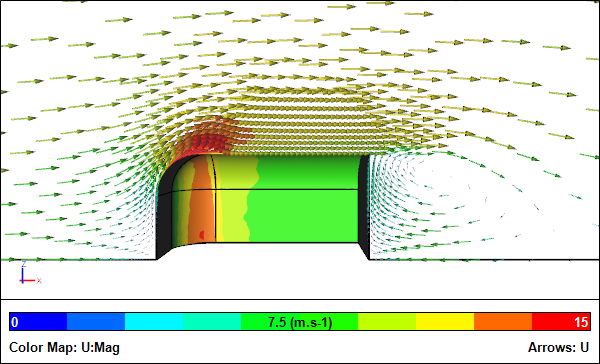CFD Simulation of a Symmetric Quarter ModelVelocity vectors

To benefit from symmetry you need to have geometric symmetry and flow symmetry. While geometric symmetry is usually easy to identify, flow symmetry is not obvious prior to running a simulation. For example, at certain Reynolds numbers the flow around a cylinder or sphere can be non-symmetric with unsteady vortex shedding.

Let's consider a simple cube with rounded edges and a blunt base that has half and quarter symmetry for comparisons. All the simulations use identical physics and solver settings in Caedium.

### Full Model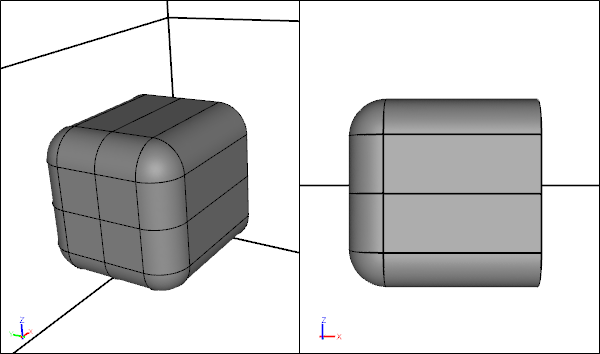Full Model Geometry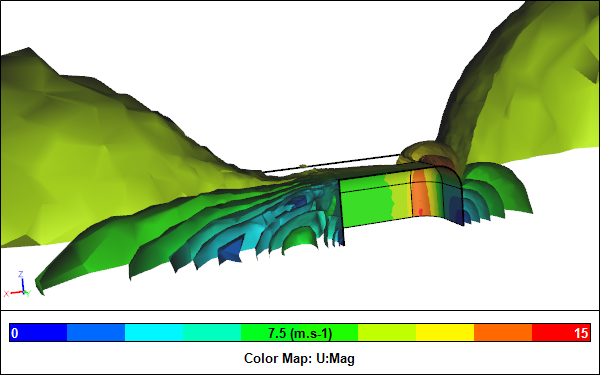Full Model CFD ResultsClipped velocity iso-surfaces

### Half Model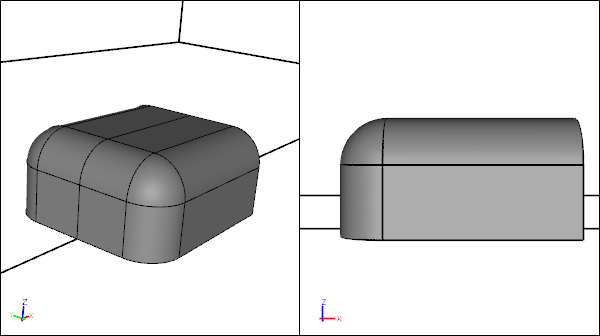Half Model Geometry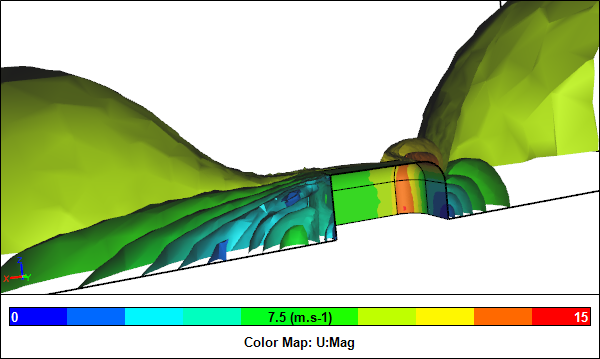Half Model CFD ResultsClipped velocity iso-surfaces

### Quarter Model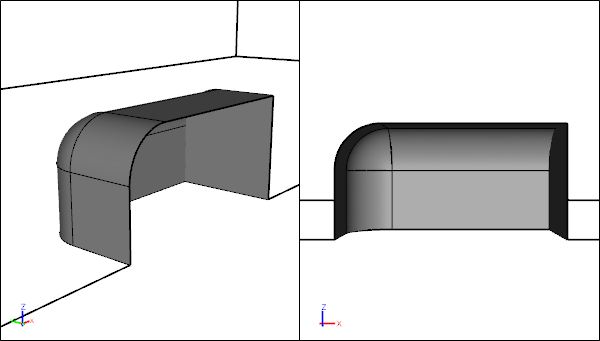Quarter Model Geometry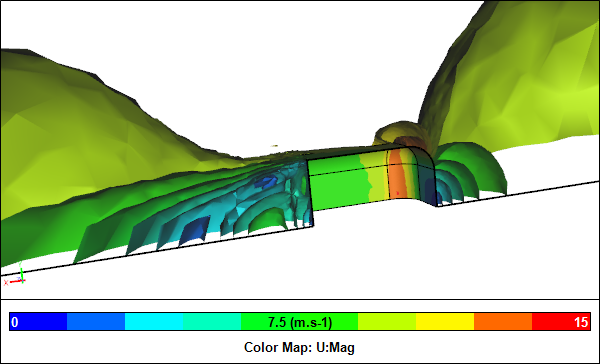Quarter Model CFD ResultsVelocity iso-surfaces

### Comparison

 Full Half Quarter Cell No. 322,249 161,761 81,866 Cell No. % 100 50 25 Node No. 59,628 30,869 16,100 Node No. % 100 52 27 Drag (N) 19.4 19.0 18.7 Drag % 100 98 96 Clock Time (s) 213 107 58 Clock Time % 100 50 27

Percentage values are relative to the full model without symmetry. The drag values for the half model where multiplied by two and the drag values for the quarter model were multiplied by four.

### Conclusion

You can see the results for all 3 cases are similar. The quarter model simulation requires 75% fewer mesh elements compared to the full model and therefore requires 75% less memory. Also the quarter model runs 73% faster than the full model simulation for the same level of convergence.

Half symmetry is typically more common than quarter symmetry and produces a 50% saving in memory and 50% faster turnaround time compared to the full model simulation. Half symmetry is especially useful for external aerodynamic simulations of cars and airplanes.

### Notes

Each model was created in Caedium Professional. The CFD simulations were performed using the incompressible, steady-state RANS solver on the dual mesh (i.e., a polyhedral mesh where the cell count is equal to the number of nodes in the original mesh), with the k-omega SST turbulence model.